LinkedList 是 Java 集合中比较常用的数据结构，与 ArrayList 一样，实现了 List 接口，只不过 ArrayList 是基于数组实现的，而 LinkedList 是基于链表实现的。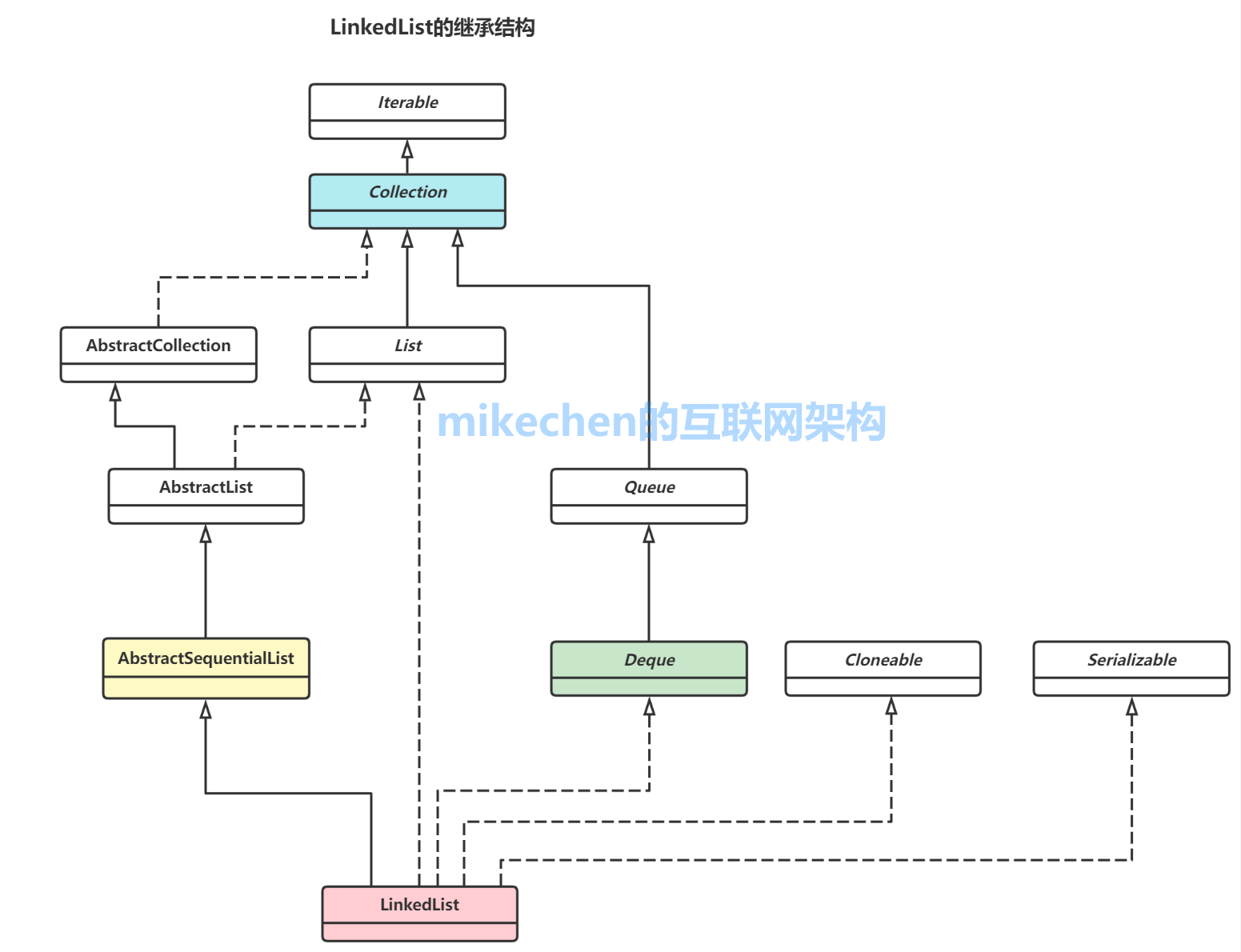```Node<E> first;
Node<E> last;```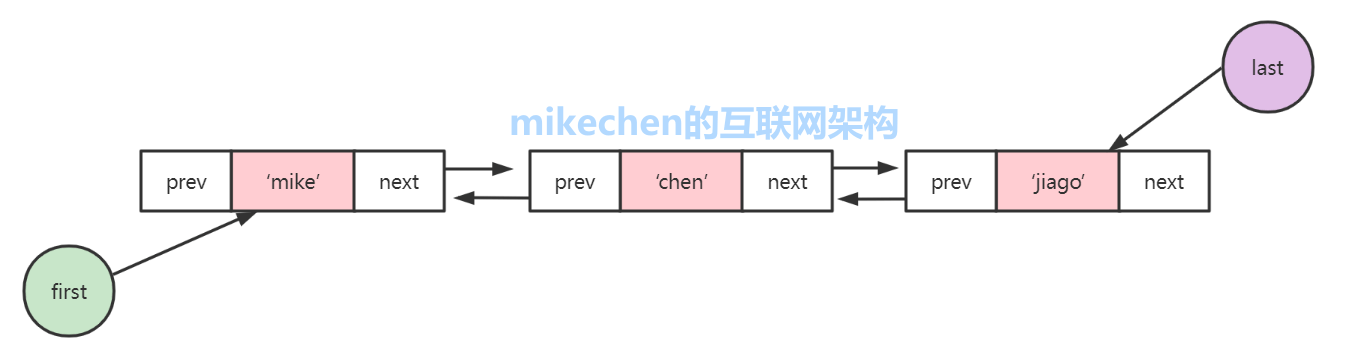```private static class Node<E> {
E item;
Node<E> next;
Node<E> prev;

Node(Node<E> prev, E element, Node<E> next) {
this.item = element;
this.next = next;
this.prev = prev;
}
}```

```// 默认构造函数

LinkedList() 仅仅构造一个空的列表，没有任何元素，size = 0，first 和 last 都为 null。

`````` transient int size = 0;
transient Node<E> first;
transient Node<E> last;
``````

``````private static class Node<E> {
E item;
Node<E> next;
Node<E> prev;

Node(Node<E> prev, E element, Node<E> next) {
this.item = element;
this.next = next;
this.prev = prev;
}
}
``````

``````@Test
}

``````public boolean add(E e) {
return true;
}

/**
* 设置元素e为最后一个元素，这个方法在添加元素到指定位置的时候也会被调用
*/
final Node<E> l = last;
// 添加e 到list 的尾部，那么此时的last 则成为e 的前置，那么e 的后置则是null
final Node<E> newNode = new Node<>(l, e, null);
last = newNode;
if (l == null)
// last 为null 意味着是空表，此时e(newNode) 则成为first
first = newNode;
else
// 否则 e(newNode) 则成为 last 的后置
l.next = newNode;
// 记录当前元素数的多少和记录修改
size++;
modCount++;
}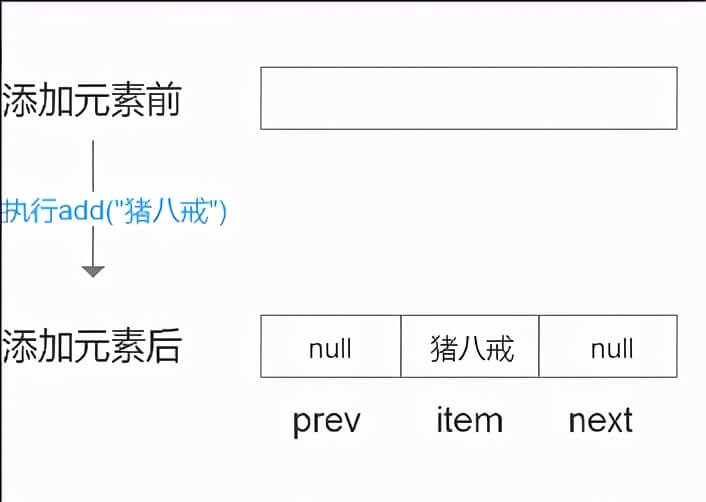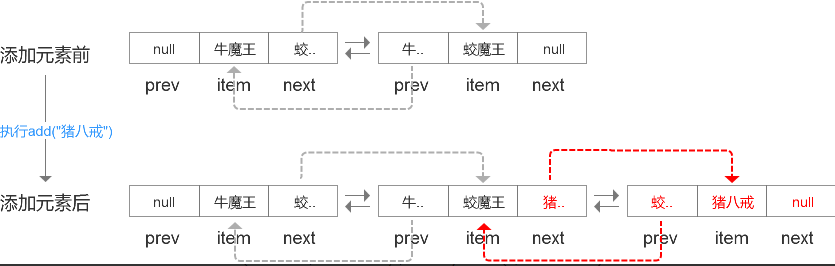### 2. add(int index, E element)

``````@Test
}

``````/**
* Inserts the specified element at the specified position in this list. Shifts the element currently at that position (if any) and any subsequent elements to the right (adds one to their indices).
* 在此列表的指定位置插入指定的元素。将当前位于该位置的元素（如果有）和任何后续元素向右移动，然后将要插入的元素进行插入
* @param index index at which the specified element is to be inserted
* @param element element to be inserted
* @throws IndexOutOfBoundsException {@inheritDoc}
*/
//在指定位置添加一个元素
public void add(int index, E element) {
// 检测下标的合法性
checkPositionIndex(index);
// 下标合法之后，则判断是不是添加到集合尾部，index=size 的时候则是添加到集合的尾部
if (index == size)
// 否则则是添加到集合的index 位置(index 不是最后一个位置)
else
// 因为不是添加到最后，所以这个里可以得到这个位置当前的元素，node(index)
}

#### checkPositionIndex

``````private void checkPositionIndex(int index) {
if (!isPositionIndex(index))
// 这个就是如果下标不合法的时候抛出的异常
throw new IndexOutOfBoundsException(outOfBoundsMsg(index));
}
/**
* Tells if the argument is the index of a valid position for an iterator or an add operation.
*/
private boolean isPositionIndex(int index) {
return index >= 0 && index <= size;
}

``````/**
* Links e as last element. 将e 作为last 元素连接起来，其实就是添加到链表末尾
*/
final Node<E> l = last;
final Node<E> newNode = new Node<>(l, e, null);
last = newNode;
if (l == null)
first = newNode;
else
l.next = newNode;
size++;
modCount++;
}

``````/**
* 在一个非空节点前插入一个元素，succ 是传入的当前位置上已经存在的元素，接下来要做的就是将e 和 succ 连接起来
*/
void linkBefore(E e, Node<E> succ) {
// assert succ != null;
// succ 的前置
final Node<E> pred = succ.prev;
// succ 作为e 的后置，succ 的前置作为e 的前置，其实就是将  succ_pree <-> succ <-> succ_next  变成  succ_pree  <-> e <->succ <-> succ_next
final Node<E> newNode = new Node<>(pred, e, succ);
succ.prev = newNode;
// 如果pree是null ,则e 要代替succ 成为新的first
if (pred == null)
first = newNode;
else
// 否则的话，e 成为succ 前置的后置
pred.next = newNode;
// 记录修改
size++;
modCount++;
}

### 3. get(int index)

``````public E get(int index) {
checkElementIndex(index);
return node(index).item;
}

checkElementIndex 方法就是在检测下标是否合法，而node 方法前面我们已经说过了，就是返回特定位置的节点，所以下面我们看一下具体是怎么检测的

``````private void checkElementIndex(int index) {
if (!isElementIndex(index))
throw new IndexOutOfBoundsException(outOfBoundsMsg(index));
}

/**
* Tells if the argument is the index of an existing element.
*/
private boolean isElementIndex(int index) {
return index >= 0 && index < size;
}

### 4. removeFirst()和removeLast()

``````/**
* Removes and returns the first element from this list. 移除list 中的第一个元素
*
* @return the first element from this list
* @throws NoSuchElementException if this list is empty
*/
public E removeFirst() {
final Node<E> f = first;
// 如果f是null 的话，则抛出异常，这里个人觉得不应该抛出异常，直接返回null 就行了，否则你每次调用这个方法的时候还得判断一下是否是空list
if (f == null)
throw new NoSuchElementException();
}

/**
* Unlinks non-null first node f. 取消first 的连接
*/
// assert f == first && f != null;
final E element = f.item;
final Node<E> next = f.next;
// help GC，为啥f 不直接赋值成null 呢
f.item = null;
f.next = null;
first = next;
if (next == null)
last = null;
else
// 因为next 已经成为first 了，应该是没有前置了
next.prev = null;
size--;
modCount++;
return element;
}

### 5. remove(Object o) 和 remove(int index)

``````//删除某个对象
public boolean remove(Object o) {
if (o == null) {
for (Node<E> x = first; x != null; x = x.next) {
if (x.item == null) {
return true;
}
}
} else {
for (Node<E> x = first; x != null; x = x.next) {
if (o.equals(x.item)) {
return true;
}
}
}
return false;
}
//删除某个位置的元素
public E remove(int index) {
checkElementIndex(index);
}
//删除某节点，并将该节点的上一个节点（如果有）和下一个节点（如果有）关联起来
final E element = x.item;
final Node<E> next = x.next;
final Node<E> prev = x.prev;

if (prev == null) {
first = next;
} else {
prev.next = next;
x.prev = null;
}

if (next == null) {
last = prev;
} else {
next.prev = prev;
x.next = null;
}

x.item = null;
size--;
modCount++;
return element;
}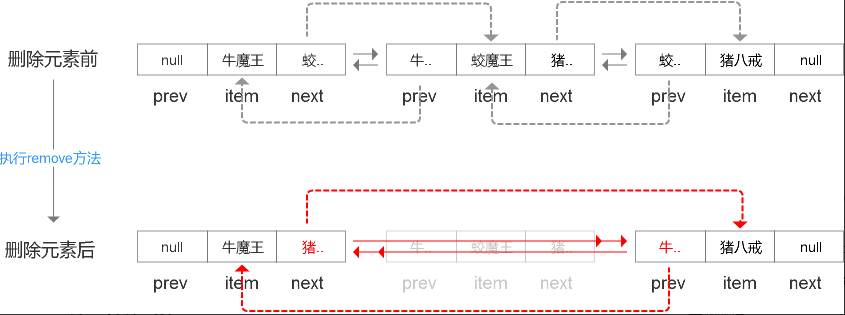``````public static void main(String[] args) {
listByNormalFor(list);
listByStrengThenFor(list);
listByIterator(list);
}

/**
* @return
*/
for (int i = 0; i < 50000; i++){
}
return list;
}

/**
*/
private static void listByNormalFor(LinkedList<Integer> list) {
// 记录开始时间
long start = System.currentTimeMillis();
int size = list.size();
for (int i = 0; i < size; i++) {
list.get(i);
}
// 记录用时
long interval = System.currentTimeMillis() - start;
System.out.println("listByNormalFor：" + interval + " ms");
}

/**
* @param list
*/
// 记录开始时间
long start = System.currentTimeMillis();
for (Integer i : list) { }
// 记录用时
long interval = System.currentTimeMillis() - start;
System.out.println("listByStrengThenFor：" + interval + " ms");
}

/**
*/
private static void listByIterator(LinkedList<Integer> list) {
// 记录开始时间
long start = System.currentTimeMillis();
for(Iterator iter = list.iterator(); iter.hasNext();) {
iter.next();
}
// 记录用时
long interval = System.currentTimeMillis() - start;
System.out.println("listByIterator：" + interval + " ms");
}

``````listByNormalFor：1067 ms
listByStrengThenFor：3 ms
listByIterator：2 ms

``````public static void listByStrengThenFor(LinkedList<Integer> list)
{
long start = System.currentTimeMillis();
Integer localInteger;
for (Iterator localIterator = list.iterator(); localIterator.hasNext();
localInteger = (Integer)localIterator.next()) {}
long interval = System.currentTimeMillis() - start;
System.out.println("listByStrengThenFor：" + interval + " ms");
}

## 作者简介说说你的看法